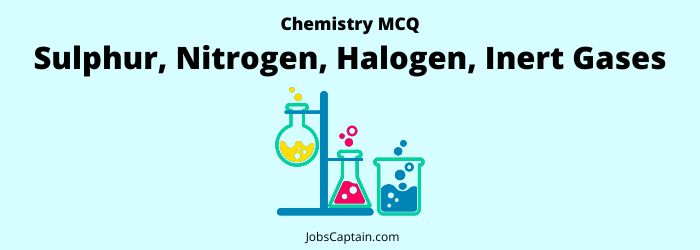# MCQ on Sulphur, Nitrogen, Halogen, Inert Gases Quiz: ChemistryTake this quiz to find out how much you know about these important elements and gases so you can be better informed about the environment around us.

Question 1. What is the shape of sulphur hexafluoride molecule?

(A) Tetrahedral

(B) Planar

(C) Octahedral

(D) Trigonal pyramid

(C) Octahedral

Question 2. Easily soluble in water?

(A) Iodine

(B) Ammonia

(C) Nitrogen

(D) Carbon

(B) Ammonia

Question 3. Which is used as Laughing gas?

(A) Nitrogen tetra oxide

(B) Nitrogen trioxide

(C) Nitrogen dioxide

(D) Nitrous oxide

(D) Nitrous oxide

Question 4. Which one of the following is also called Stranger Gas?

(A) Nitrous Oxide

(B) Xenon

(C) Neon

(D) Argon

(B) Xenon

Question 5. Which one of the following is not correctly matched?

(A) Nitric oxide is a laughing gas

(B) Dry ice is solid Carbon dioxide

(C) Boric acid is used in pharmaceutical industry and also as a food preservative

(D) Bordeaux mixture is a mixture of Copper (II) Sulphate and Calcium Hydroxide in water and is used as a fungicide

(A) Nitric oxide is a laughing gas

Question 6. Discharge of Nitrogen causes?

(A) Increase in the amount of atmospheric nitrogen

(B) Increase in the amount of Nitrogen in lithosphere

(C) The amount of Nitrogen in lithosphere and in atmosphere unchanged

(D) Destruction of nitrates bacteria

(C) The amount of Nitrogen in lithosphere and in atmosphere unchanged

Question 7. The gas used to inflate the tyres of an aircraft is ________.

(A) Neon

(B) Helium

(C) Nitrogen

(D) Hydrogen

(C) Nitrogen

Question 8. In order to compensate the deficiency of which of the following elements, the insectivorous plants trap and eat insects?

(A) Sulphur

(B) Magnesium

(C) Nitrogen

(D) Manganese

(C) Nitrogen

Question 9. The soil in which insectivorous plants grow is deficient in ____________.

(A) Magnesium

(B) Calcium

(C) Nitrogen

(D) Water

(C) Nitrogen

Question 10. Which gas is usually filled in the balloon?

(A) Helium

(B) Carbon dioxide

(C) Oxygen

(D) Hydrogen

(A) Helium

Question 11. Helium is preferred to Hydrogen in air balloons because it ______________.

(A) Does not form an explosive mixture with air

(B) Has greater lifting power

(C) Is less dense

(D) Is cheaper

(A) Does not form an explosive mixture with air

Question 12. Tear gas is __________.

(A) Hydrogen Sulphide

(B) Hydrogen Carbide

(C) Chlorine

(D) Ammonia

(D) Ammonia

Question 13. Which of the following gas is used as tear gas?

(A) Cl2

(B) NH3

(C) SO2

(D) H2

(B) NH3m

Question 14. The foul smell of dead fish is due to the compound?

(A) Nitro compounds

(B) Sulphur compounds

(C) Aldehydic compounds

(D) Amino compounds

(D) Amino compounds

Question 15. Which of the following remains in a solid state at normal temperature?

(A) Fluorine

(B) Iodine

(C) Bromine

(D) Chlorine

(B) Iodine

Question 16. Which of the following is not optically active?

(A) All of these

(B) Serine

(C) Alanine

(D) Glycine

(D) Glycine

Question 17. The most reactive among the Halogens is _____________.

(A) Fluorine

(B) Chlorine

(C) Bromine

(D) Iodine

(D) Iodine

Question 18. The halogen being used as analgesic is ___________.

(A) Fluorine

(B) Iodine

(C) Bromine

(D) Chlorine

(C) Bromine

Question 19. Which has is filled at low pressuren in a tube light?

(A) Only Argon

(B) Nitrogen and Neon

(C) Neon and Mercury vapour

(D) Argon and Neon

(C) Neon and Mercury vapour

Question 20. Tubelight is filled with ___________.

(A) Sodium vapour

(B) Argon gas at low pressure

(C) Mercury vapour at low pressure

(D) Both (B) and (C)

(D) Both (B) and (C)

Question 21. Which one of the following mixture of gas is used by the deep sea divers?

(A) Mixture of Oxygen-Hydrogen

(B) Mixture of Oxygen-Acetylene

(C) Mixture of Helium-Nitrogen

(D) Mixture of Helium-Oxygen

(D) Mixture of Helium-Oxygen

Question 22. Which gas is mixed with Oxygen for respiration by divers in deep sea?

(A) Ammonia

(B) Xenon

(C) Nitrogen

(D) Helium

(D) Helium

Question 23. Percentage amount (by volume) of which one of the following gas is lowest in the atmosphere?

(A) Oxygen

(B) Nitrogen

(C) Carbon dioxide

(D) Argon

(C) Carbon dioxide

Question 24. Which gas is known as ‘noble gas’?

(A) Carbon dioxide

(B) Helium

(C) Oxygen

(D) Hydrogen

(B) Helium

Question 25. Which gas is not in the gaseous cycle?

(A) H2

(B) Carbon

(C) O2

(D) N2

(A) H2

Question 26. What is the approximate percentage of Nitrogen present in the atmosphere?

(A) 78-79%

(B) 40-42%

(C) 18-20%

(D) 10-11%